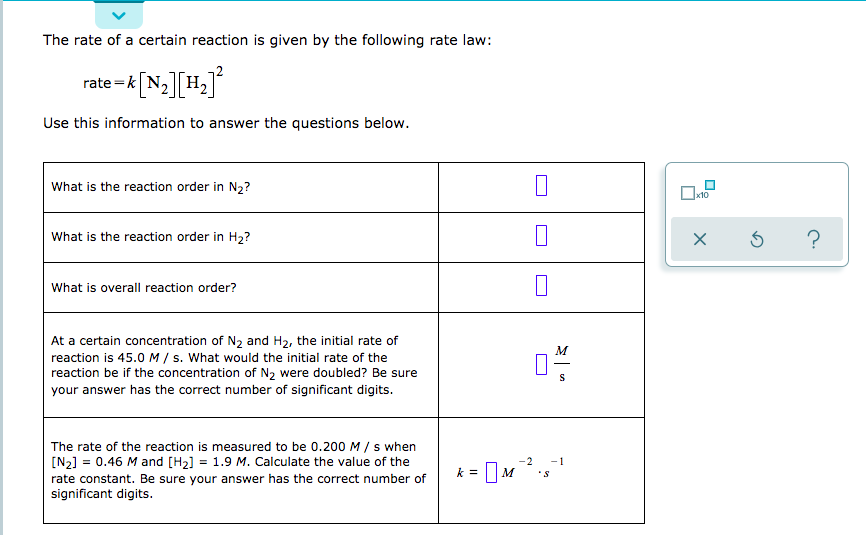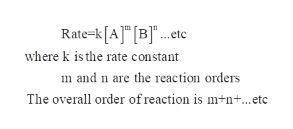# The rate of a certain reaction is given by the following rate law:rate-k N2 H2Use this information to answer the questions below.What is the reaction order in N2?10What is the reaction order in H2?What is overall reaction order?At a certain concentration of N2 and H2, the initial rate ofMreaction is 45.0 M / s. What would the initial rate of thereaction be if the concentration of N2 were doubled? Be sureyour answer has the correct number of significant digits.The rate of the reaction is measured to be 0.200 M/ s when[N2] 0.46 M and [H2] = 1.9 M. Calculate the value of the-21=rate constant. Be sure your answer has the correct number ofsignificant digits

Question
58 views

I just need help with the last 2 questions with correct significant digitshelp_outlineImage TranscriptioncloseThe rate of a certain reaction is given by the following rate law: rate-k N2 H2 Use this information to answer the questions below. What is the reaction order in N2? 10 What is the reaction order in H2? What is overall reaction order? At a certain concentration of N2 and H2, the initial rate of M reaction is 45.0 M / s. What would the initial rate of the reaction be if the concentration of N2 were doubled? Be sure your answer has the correct number of significant digits. The rate of the reaction is measured to be 0.200 M/ s when [N2] 0.46 M and [H2] = 1.9 M. Calculate the value of the -2 1 = rate constant. Be sure your answer has the correct number of significant digits fullscreen
check_circle

star
star
star
star
star
1 Rating
Step 1

Given:

Rate=k (N2) (H2)2

Initial rate of reaction=45.0 M/s

Rate of reaction=0.200 M/s

Molarity of N2 =0.46 M

Molarity of H2 =1.9 M

Step 2

The rate law has the form:help_outlineImage TranscriptioncloseRate-k[A] [B etc where k is the rate constant m and n are the reaction orders The overall order of reaction is m+n...etc fullscreen
Step 3

The rate of a certai...

### Want to see the full answer?

See Solution

#### Want to see this answer and more?

Solutions are written by subject experts who are available 24/7. Questions are typically answered within 1 hour.*

See Solution
*Response times may vary by subject and question.
Tagged in

### Chemistry